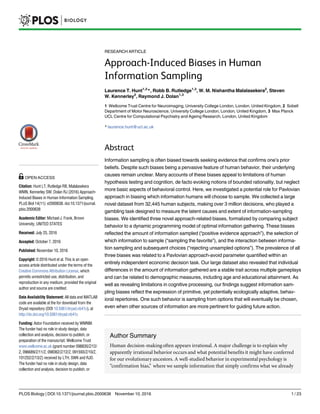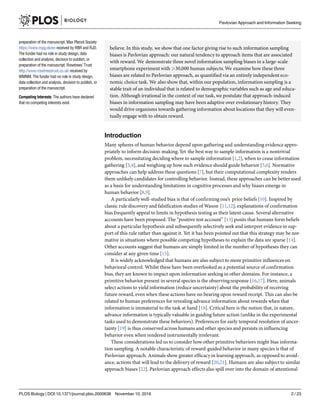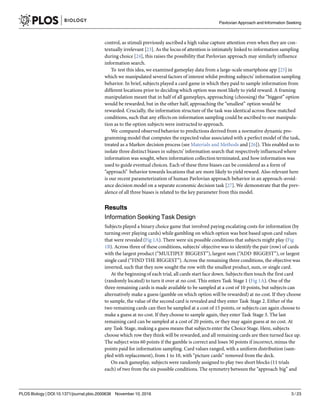Ce diaporama a bien été signalé.
Le téléchargement de votre SlideShare est en cours. ×

# Journal.pbio.2000638

Publicité
Publicité
Publicité
Publicité
Publicité
Publicité
Publicité
Publicité
Publicité
Publicité
PublicitéPublicité
Publicité
Publicité
Publicité
Chargement dans…3
×

## Consultez-les par la suite

1 sur 23 Publicité

# Journal.pbio.2000638

Article Source: Approach-Induced Biases in Human Information Sampling
Hunt LT, Rutledge RB, Malalasekera WMN, Kennerley SW, Dolan RJ (2016) Approach-Induced Biases in Human Information Sampling. PLOS Biology 14(11): e2000638. https://doi.org/10.1371/journal.pbio.2000638

Article Source: Approach-Induced Biases in Human Information Sampling
Hunt LT, Rutledge RB, Malalasekera WMN, Kennerley SW, Dolan RJ (2016) Approach-Induced Biases in Human Information Sampling. PLOS Biology 14(11): e2000638. https://doi.org/10.1371/journal.pbio.2000638

Publicité
Publicité

### Journal.pbio.2000638

6. 6. As expected, the dynamic programming model predicts identical behavior irrespective of the subject’s approach goal. As an example, consider revealing a 2 in the MULTIPLY BIG con- dition on an AA trial. This yields a probability of 0.764 that row B will be rewarded, and the expected value of guessing is therefore 0.764 Ã 60 + (1–0.764) Ã (-50) = 34. The expected value of sampling again from the A row, calculated using dynamic programming, is 28.8, and so the relative expected value of guessing is 5.2 (Fig 2A). Now consider seeing a 2 in the MULTIPLY SMALL condition. This now yields the exact same probability of 0.764 that row A will be rewarded. Hence the expected value of guessing remains 34. The expected value of sampling further information remains 28.8, and so the relative expected value of guessing remains 5.2. In contrast with these model predictions, subjects’ actual behavior showed a systematic dif- ference between MULTIPLY BIG and MULTIPLY SMALL conditions (compare circles and plus signs in Fig 2B, see also S1 Fig). Subjects became more likely to guess if they had seen evi- dence that supported them approaching row A rather than avoiding it. In MULTIPLY BIG, a high-valued card (6 or above) carries evidence for choosing row A. Subjects become more likely to guess than when seeing the same card in MULTIPLY SMALL. However, a low-valued card in MULTIPLY BIG (5 or below) carries evidence for avoiding row A. Subjects now become more likely to sample than when seeing the same card in MULTIPLY SMALL. This framing effect is seen most clearly when subtracting behavior in MULTIPLY SMALL from MULTIPLY BIG (Fig 2C). The observedbias is one of approaching an option if positive evidence has been provided in support of that option; consequently, we term this “positive evidence approach.” We observed that positive evidence approach was more pronounced in AB trials than AA trials (Fig 2C). This is again consistent with our hypothesis, as subjects are less inclined to sample further information if it is available on a row they wish to avoid than if it is on a row they wish to approach. To quantify positive evidence approach across our population, we used the following sum- mary statistic: X10 i¼6 ðpGuessbig;i À pGuesssmall;iÞ þ X5 i¼1 ðpGuesssmall;i À pGuessbig;iÞ where pGuessbig,i and pGuesssmall,i denote the average probability of guessing in MULTIPLY BIG and MULTIPLY SMALL, respectively, having revealed card value i. As there should be no difference in the probability of guessing across the two conditions, the expected value of this statistic from the normative model is 0. By contrast, the value of this statistic across our popula- tion was 0.42 in AA trials and 0.70 in AB trials. To estimate our confidence in this summary statistic, we recomputed it on 1,000 bootstrapped samples of 10,000 gameplays from our popu- lation. This yielded 95% confidence intervals of [0.30, 0.54] in AA trials and [0.62, 0.79] in AB trials. (Throughout the paper, we focus on the reporting of effect sizes and 95% confidence intervals rather than p-values, as our large sample size renders p-values less informative .) Similar results are seen by comparing the ADD BIG and ADD SMALL conditions (S2 Fig; AA trials: mean 0.52, 95% CIs [0.40, 0.64]; AB trials: mean 0.79, 95% CIs [0.70, 0.89]). See also S3 Fig for FIND THE BIGGEST/FIND THE SMALLEST, which are not directly matched for information content. It is also notable that, overall, subjects’ behavioral choices to sample information were simi- lar to predictions arising from the optimal model (Fig 2B), although not identical (S1 Fig). This alone does not imply that subjects are implementing the optimal model. Instead, it may simply reflect the fact that relatively simple behavioral strategies will often recapitulate many features of more sophisticated strategies . For example, one straightforward strategy would be to Pavlovian Approach and Information Seeking PLOS Biology | DOI:10.1371/journal.pbio.2000638 November 10, 2016 6 / 23
9. 9. BIG (Fig 4C). Whereas the optimal model shows no difference between these two conditions (i.e., the entire heat map should equal 0), subjects reliably sampled information from the row that they currently sought to approach rather than avoid. We term this bias “sampling the favorite.” To quantify sampling the favorite, we derived two statistics for trials in which subjects decided to sample a third piece of information. We cal- culated one “strong evidence” statistic for trials in which the “favorite” (the item that would eventually be approached) was clear. We defined this as trials where the difference in card val- ues was 4 or greater in magnitude: X10 i¼5 XiÀ 4 j¼1 ðpðSample ¼ AÞi;j;big À pðSample ¼ AÞi;j;smallÞÀ X10 j¼5 XjÀ 4 i¼1 ðpðSample ¼ AÞi;j;big À pðSample ¼ AÞi;j;smallÞ where P(Sample = A)i,j,big refers to the relative probability of choosing to sample from row A Fig 4. Sampling the favorite bias at Task Stage 2. If subjects choose to sample at Task Stage 1, they enter Task Stage 2. If this is on an AB trial, they then may sample again from row A, or from row B, or make a guess. (A) Model predictions. The relative expected value (in points) of sampling from row A versus sampling from row B in the MULTIPLY conditions. It is generally more valuable to sample from the row that currently has the higher-valued card (e.g., the 7, in the example shown). Crucially, this prediction is the same in both MULTIPLY BIG and MULTIPLY SMALL conditions. (B) Subject behavior. Subjects show a propensity to sample from the row containing the high-valued card in MULTIPLY BIG, but this trend is reversed in MULTIPLY SMALL. Subjects are therefore inclined to sample the option that is currently most likely to be approached. (C) Sampling the favorite bias is revealed by subtracting MULTIPLY SMALL trials from MULTIPLY BIG trials. The normative model predicts this heat map to show no difference between conditions, yet there is a clear tendency to sample the currently favored row. See also S6 Fig and S7 Fig for other conditions, and S8 Fig for relative value of guessing versus sampling across all six conditions. Data for reproducing all analyses is freely available for download from Dryad. doi:10.1371/journal.pbio.2000638.g004 Pavlovian Approach and Information Seeking PLOS Biology | DOI:10.1371/journal.pbio.2000638 November 10, 2016 9 / 23
10. 10. over row B, when card i is presented on row A and card j presented on row B, on MULTIPLY BIG trials. The top row of the equation denotes trials where row A has a higher-valued card than row B, favoring approaching A in MULTIPLY BIG but approaching B in MULTIPLY SMALL. The converse is true for the bottom row. We calculated a second “weak evidence” statistic for trials in which the “favorite” was less clear. We defined this as trials in which the difference in card values was between 1 and 3 in magnitude: X10 i¼2 XiÀ 1 j¼maxð1;iÀ 3Þ ðpðSample ¼ AÞi;j;big À pðSample ¼ AÞi;j;smallÞÀ X10 j¼2 XjÀ 1 i¼maxð1;jÀ 3Þ ðpðSample ¼ AÞi;j;big À pðSample ¼ AÞi;j;smallÞ Crucially, because the optimal model predicts identical values for sampling from row A versus row B on MULTIPLY BIG and MULTIPLY SMALL, the expected value for both statistics is always 0. In contrast, the value of the “strong evidence” statistic across our population was 12.35 (95% CIs [10.55, 14.01]), whilst the value of the “weak evidence” statistic was 7.21 (95% CIs [5.85, 8.48]). Note this bias was also observedin the ADD BIG/ADD SMALL condition (S6 Fig), where the value of the “strong evidence” statistic was 11.93 (95% CIs [10.39, 13.51]), whilst the value of the “weak evidence” statistic was 6.86 (95% CIs [5.61, 8.14]). See S7 Fig for FIND THE BIG- GEST/FIND THE SMALLEST, which are not directly matched for information content. A Parametric Model of Subject Behavior We consider that subjects are unlikely to be implementing dynamic programming when they perform the task, yet their overall behavior shows a surprising resemblance to model predic- tions (e.g., Fig 2B, Fig 3B). We therefore constructed a simpler model that describes subjects’ performance without recourse to dynamic programming. In this model, subjects first compute the value of choosing each option by comparing the presented value to the average value of all possible cards. In ADD BIG trials, at stage 1, for example, this would be the following: VðAÞ ¼ b1ð1stCardValueÀ < 1stCardValue >Þ VðBÞ ¼ b1ð< 1stCardValue > À 1stCardValueÞ where <1stCardValue> is the expected value of the first card (5.5) and β1 is a free parameter. In ADD SMALL trials, we simply inverted the value of the each card, such that 10 became 1, 9 became 2, and so on. We also considered an AB trial (e.g., Fig 4B), where after turning the second card, the values of option A and B become the following: VðAÞ ¼ b1ð1stCardValue À 2ndCardValueÞ VðBÞ ¼ b1ð2ndCardValue À 1stCardValueÞ Pavlovian Approach and Information Seeking PLOS Biology | DOI:10.1371/journal.pbio.2000638 November 10, 2016 10 / 23
11. 11. At both stages, we compute the degree of uncertainty, ω, in choosing either option: o ¼ À ð 1 1 þ eVðBÞÀ VðAÞ À 0:5Þ 2 This is then used to derive the value of sampling information from option A or option B: Vðsample AÞ ¼ b2 þ b3 o Vðsample BÞ ¼ b2 þ b3 o The probability of each choice C being option o is finally generated using a softmax choice rule: p C ¼ oð Þ ¼ e VðoÞ t P ie VðiÞ t where i indexes the entire set of available options at a given stage of the task. Hence, at stage 1 of an AA trial, it indexes the set {choose A, choose B, sample A}; at stage 1 of an AB trial, it indexes the set {choose A, choose B, sample B}; at stage 2 of an AB trial, it indexes the set {choose A, choose B, sample A, sample B}. Note that the model therefore assumes a three- or four-way decision at each stage of the game. Although in the structure of the task this was made as two sequential binary decisions (guess or sample, then select a row), it reflects the intu- ition that when subjects decide to guess, they already have internally committed to choosing a particular row. We fit parameters β1, β2, β3, and τ, using maximum likelihoodestimation (fminsearch in MATLAB) separately at stage 1 and stage 2. Fitting was repeated at 50 random seed locations to avoid local minima, and the model was fit to the average behavior of all subjects across each condition using a sum of squared errors cost function. We performed fitting separately for both ADD and MULTIPLY conditions; we do not consider FIND THE BIGGEST/SMALLEST conditions here, as these are not matched for information content. Note that this model does not explicitly feature terms for the costs associated with sampling information; instead, these are implicitly factored into the constant term β2. This model captures the main features of the behavioral data (S9 Fig). At stage 1, it displays a U-shaped effect of card value on information sampling (as in Fig 2B) caused by the effects of choice uncertainty on the value of sampling information. It also displays a softmax choice curve (as in Fig 3B) between options A and B, matching subjects’ real choice probabilities between these two options. At stage 2, it displays choice probabilities between options A and B that again closely match subjects’ behavior. However, because this model makes symmetric predictions for BIG and SMALL trials, it fails to capture the three biases describedabove (S9 Fig). We therefore adapted the model with three additional parameters to capture these biases (Fig 5). At stage 1, before entering values into the softmax choice equation, we captured the “rejecting unsampled options” bias (Fig 5D– 5F) by adding an “approach bonus” (β4) to the value of the item that could not be sampled. This makes subjects more likely to choose this option if they do not sample information. VðAÞ ¼ VðAÞ þ b41stCardValue ½on AB trials only; VðBÞ ¼ VðBÞ þ b4 < 1stCardValue > ½on AA trials only: A natural consequence of this value modulation by β4 is that it also induces a change in the form of the uncertainty ω, which determines the value of sampling information (see above). Whereas previously this would have been symmetric around the average value of the first card, Pavlovian Approach and Information Seeking PLOS Biology | DOI:10.1371/journal.pbio.2000638 November 10, 2016 11 / 23
12. 12. Fig 5. Behavioral predictions from the full parametric model of subject behavior, with best-fit parameters. (A) Predicted probability of guessing at stage 1 for AA trials (compare to Fig 2Bi) and (B) AB trials (compare to Fig 2Bii) in “add” condition. (C) Predicted “positive evidence approach” bias. Compare to Fig 2C. (D) Predicted probability of choosing row A, having chosen to guess, at stage 1, for “add big” (compare to Fig 3Bi) and (E) “add small” (compare to Fig 3Bii) conditions. (F) Predicted “rejecting unsampled options” bias. Compare to Fig 3C. (G) Predicted probability of sampling row A in “big” (compare to Fig 4Bi) and in (H) “small” (compare to Fig 4Bii) conditions. (I) Predicted “sampling the favorite” bias. Compare to Fig 4C. See also S9 Fig. Data for reproducing all analyses is freely available for download from Dryad. doi:10.1371/journal.pbio.2000638.g005 Pavlovian Approach and Information Seeking PLOS Biology | DOI:10.1371/journal.pbio.2000638 November 10, 2016 12 / 23